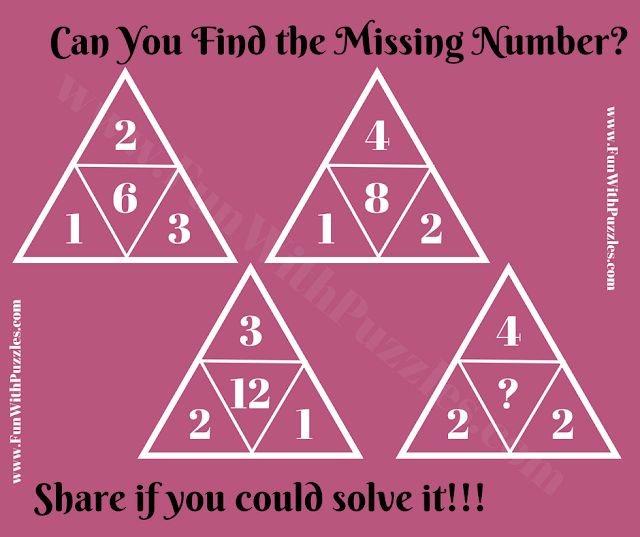If you're seeking a stimulating math logic challenge, you've come to the right place. Our Fun Math Triangle Number Puzzle is designed to engage and invigorate your mathematical reasoning. Unlike ordinary puzzles, this one requires more than just adding and subtracting numbers; it demands a keen eye for patterns and mathematical relationships.

Here's how it works: You're presented with four triangles, each containing a number. These numbers aren't random; they're interconnected through a concealed mathematical equation or pattern. Your mission? Unearth this hidden pattern and unveil the missing number represented by the question mark. It's a cerebral exercise that promises to enhance your number-solving prowess.

Are you ready to embrace this mathematical challenge? Solving puzzles like this can sharpen your mind, improve logical thinking, and provide hours of intellectual stimulation. So go ahead, tackle the Math Triangle Puzzle, and see if you can crack the code to reveal the solution.Number Riddle: Can you find the Missing Number?

The answer to this "Fun Math Triangle Puzzle for Adults", can be viewed by clicking the button. Please do give your best try before looking at the answer.